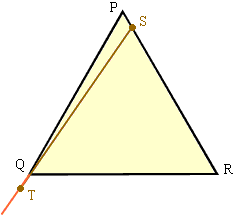Quandaries and Queries In a triangle i have the length of a line and it's opposite angle.how can i calculate perimeter?(the angles are not right) Hi, There is not enough information here to calculate the perimeter. Let me illustrate with an example. I am going to use an equilateral triangle PQR with all sides of length 1 so the angle RPQ is 60o.Let S be a point on the line segment PR which is very close to P. Extend the line segment PQ. Let T be the point on PQ extended such that the length of ST is 1, that is |ST| = 1. PQR and PTS are two triangles. Each has a side of length 1, QR and TS, and the angle opposite this side is 60o in each triangle. The perimeter of PQR is 1+1+1=3. The perimeter of PTS is |PT| + |TS| + |SP| = |PQ| + |QT| + |TS| + |SP| = 1 + |QT| + 1 + |SP| = 2 + |QT| + |SP| But |QT| and |SP| are both very small, in fact you can make them as small as you want by taking S very close to P. Thus you can ensure that |QT|+|SP| is less than 1 and hence the perimeter of PTS is less than 3. Penny Go to Math Central